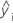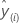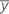# Methods and formulas for model selection in Partial Least Squares Regression

Select the method or formula of your choice.

## R-sq

R2 is also known as the coefficient of determination.

### Notation

TermDescription
yi i th observed response valuemean responsei th fitted response

## SS

The sum of the squared distances. SS Regression is the portion of the variation explained by the model. SS Error is the portion not explained by the model and is attributed to error. SS Total is the total variation in the data.

SS Regression:
SS Error:
SS Total:

### Notation

TermDescription
yi i th observed response valuei th fitted responsemean response

## PRESS

The prediction sum of squares (PRESS) statistic assesses your model's predictive ability. PRESS, similar to the residual sum of squares, is the sum of squares of the prediction error. In PLS, Minitab only calculates PRESS if you cross-validated the model.

Minitab calculates PRESS in the following steps:

1. Minitab recalculates the model as many times as there are observations, omitting a different observation each time. For each omitted observation, Minitab calculates the fitted or predicted response using the model.
2. Minitab subtracts the predicted value from the observed response value. This is the true prediction error because the observation fit is independent of the model.
3. Once Minitab conducts this routine for all observations, Minitab calculates PRESS using the formula:

In general, the smaller the PRESS value, the better the model's predictive ability. PRESS is used to calculate the predicted R2.

### Notation

TermDescription
yithe observed responsethe fitted response for the omitted observation
nthe number of observations

## R-sq (pred)

While the calculations for R2(pred) can produce negative values, Minitab displays zero for these cases.

### Notation

TermDescription
yi i th observed response valuemean response
n number of observations
ei i th residual
hi i th diagonal element of X(X'X)–1X'
X design matrix

## Test R-sq

Indicates how well the PLS model predicts your test data. The test R2 represents the proportion of variation in the responses that is explained by the predictors in your test data set. Generally, test data is used to validate the fitted model and must include the same number of predictors as the original data set. The test R2 can only be calculated if the test data includes response data for each observation. The test R2 is calculated in the same way as R2 with this formula:
By using this site you agree to the use of cookies for analytics and personalized content.  Read our policy